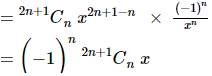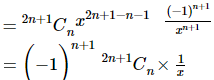Find the middle term in the expansion of $$(x-\dfrac{ 1}{x})^{2n+1}$$

Asked by Sakshi | 1 year ago |  338

##### Solution :-

where, n = (2n + 1) is an (odd number)

So the middle terms are $$\dfrac{(n+1)}{2}$$

= $$\dfrac{ (2n+1+1)}{2}$$

=$$\dfrac{ (2n+2)}{2}$$ = (n + 1) and

$$\dfrac{(n+1)}{2} + 1$$

=$$\dfrac{ (2n+1+1)}{2 + 1}$$

= (n + 1 + 1) = (n + 2)

The terms are (n + 1)th and (n + 2)th.

Now,

Tn = Tn+1And,

Tn+2 = Tn+1+1Hence, the middle term is (-1)n.2n+1Cn x and (-1)n+1.2n+1Cn ($$\dfrac{1}{x}$$).

Answered by Sakshi | 1 year ago

### Related Questions

#### Find the term independent of x in the expansion of (3/2 x2 – 1/3x)9

Find the term independent of x in the expansion of $$(\dfrac{3}{2x^2} – \dfrac{1}{3x})^9$$

#### Find the middle term in the expansion of (1 + 3x + 3x2 + x3)2n

Find the middle term in the expansion of (1 + 3x + 3x2 + x3)2n

#### Find the middle term in the expansion of (1 – 2x + x2)n

Find the middle term in the expansion of (1 – 2x + x2)n

Find the middle term in the expansion of $$(\dfrac{x}{a} – \dfrac{a}{x})^{10}$$
Find the middle term in the expansion of $$(x^2 – \dfrac{2}{x})^{10}$$